Examples

Chapter 4 Class 10 Quadratic Equations (Term 2)
Serial order wise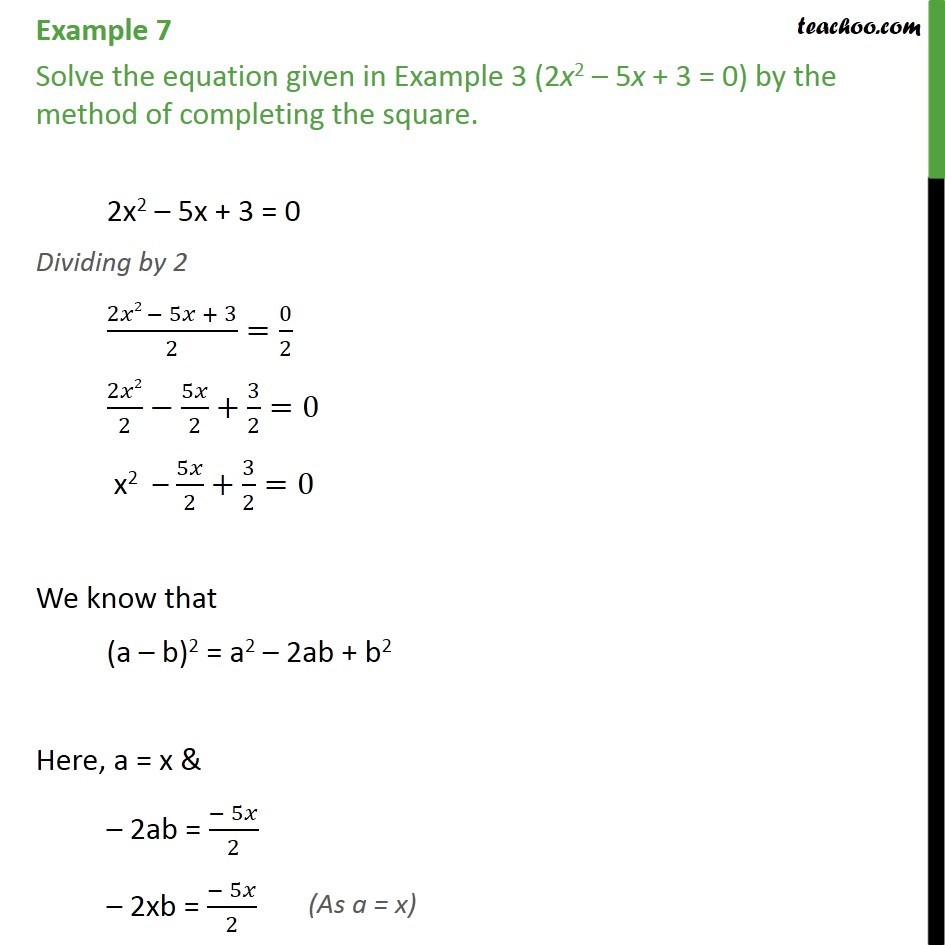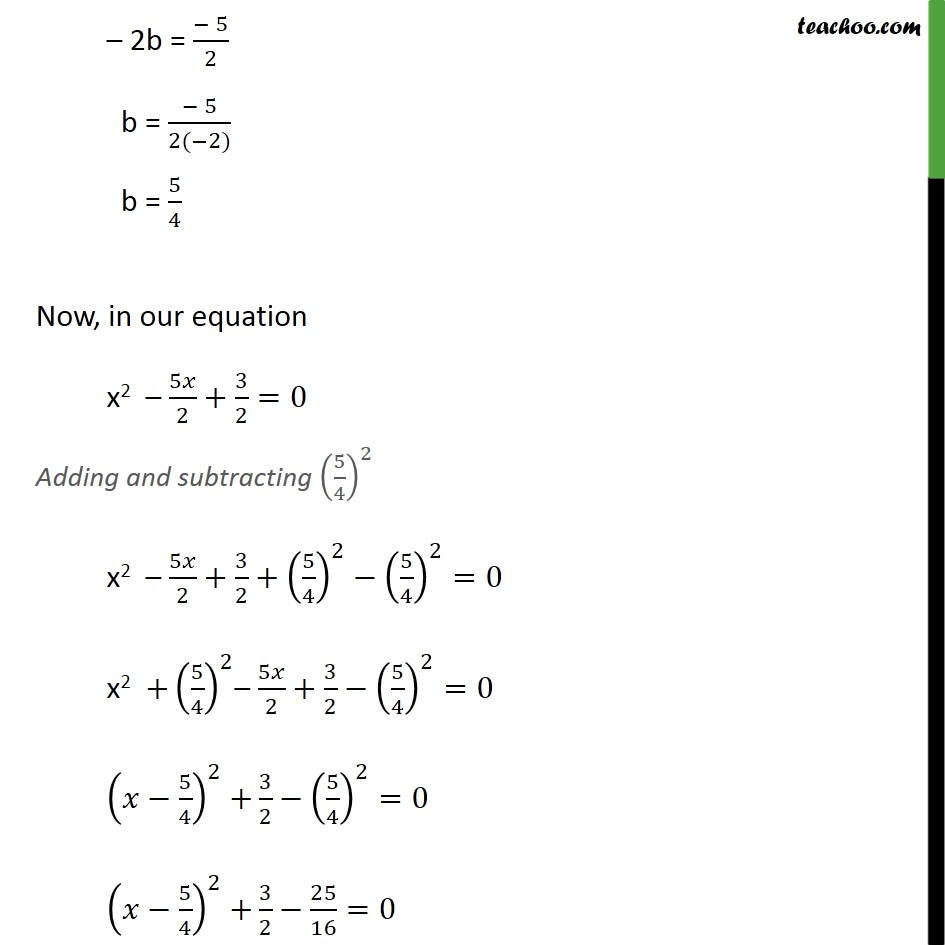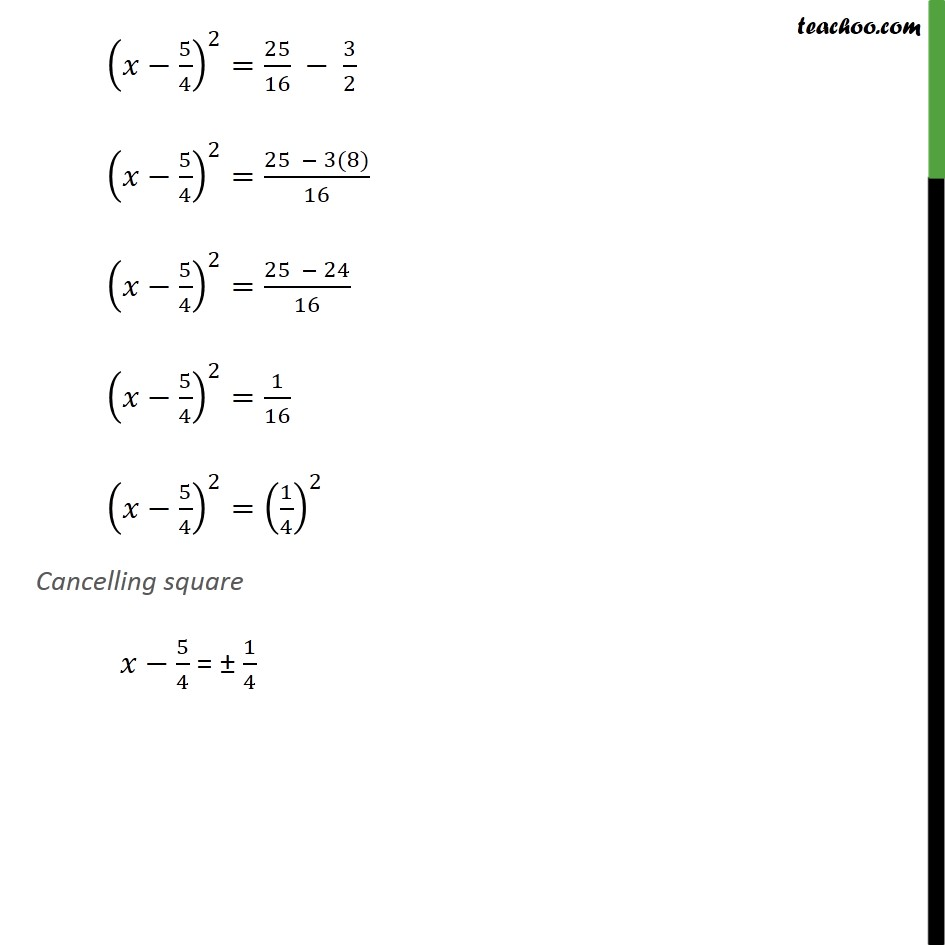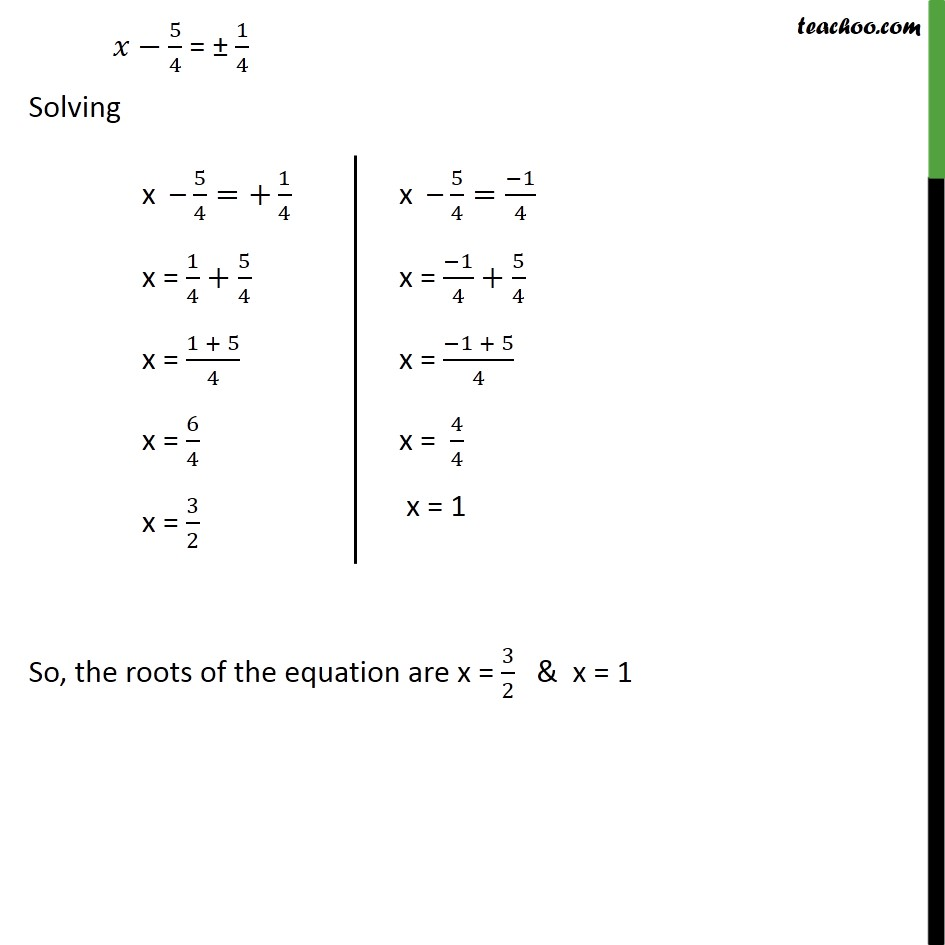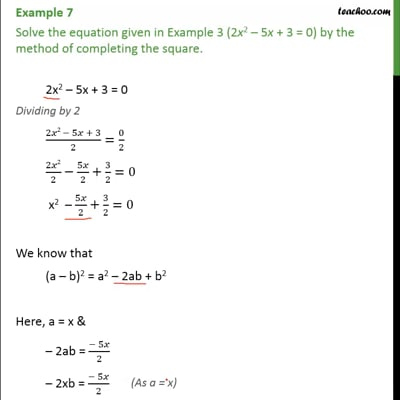This video is only available for Teachoo black users

### Transcript

Example 7 Solve the equation given in Example 3 (2x2 5x + 3 = 0) by the method of completing the square. 2x2 5x + 3 = 0 Dividing by 2 (2 2 5 + 3)/2=0/2 2 2/2 5 /2+3/2=0 x2 5 /2+3/2=0 We know that (a b)2 = a2 2ab + b2 Here, a = x & 2ab = ( 5 )/2 2xb = ( 5 )/2 2b = ( 5)/2 b = ( 5)/(2( 2)) b = 5/4 Now, in our equation x2 5 /2+3/2=0 Adding and subtracting (5/4)^2 x2 5 /2+3/2+(5/4)^2 (5/4)^2=0 x2 +(5/4)^2 5 /2+3/2 (5/4)^2=0 ( 5/4)^2 +3/2 (5/4)^2=0 ( 5/4)^2 +3/2 25/16=0 ( 5/4)^2=25/16 3/2 ( 5/4)^2=(25 3(8))/16 ( 5/4)^2=(25 24)/16 ( 5/4)^2=1/16 ( 5/4)^2=(1/4)^2 Cancelling square 5/4 = 1/4 5/4 = 1/4 Solving So, the roots of the equation are x = 3/2 & x = 1